ISEE Middle Level Math : Numbers and Operations

Example Questions

Example Question #11 : Ratio And Proportion

In March, there were 8 rainy days, 13 sunny days and 10 cloudy days. What is the ratio of sunny days to total days in March?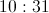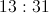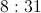Explanation:

Identify the number of sunny days in March:Create a ratio showing sunny days in March to toal days in March (31 day month):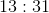Example Question #11 : Ratio And Proportion

A 32-ounce bottle of Emerald Cola costs $1.39. What is the cost per ounce, taken to the nearest tenth of a cent? Possible Answers: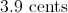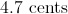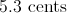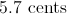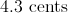Correct answer:Explanation: To find the cost per ounce, divide the total cost,$1.39, by the number of ounces, 32.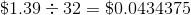This rounds to 4.3 cents per ounce.

Example Question #13 : Ratio And Proportion

A batting average is defined as the ratio of hits to turns at bat. It is expressed as a decimal rounded to the nearest thousand.

In 2013, Carlos Beltran's batting average was 0.296. If he maintains this same batting average over the 2014 baseball season, how many turns at bat should he expect to need to achieve 40 hits? (Nearest whole number)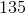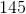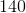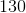Explanation:

Since a batting average is a ratio of hits to turns at bat,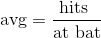Letbe the number of turns at bat Beltran needs to get 40 hits. Then solve forin this equation: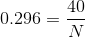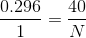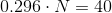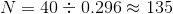Beltran should need 135 turns at bat.

Example Question #12 : Numbers And Operations

A furlong is a unit of length used in horse racing. Eight furlongs are equal to one mile. How many feet are equal to twelve furlongs?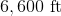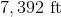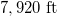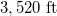Explanation:

Multiply 12 furlongs by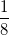miles per furlong, and by 5,280 feet per mile: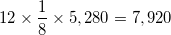feet.

Example Question #13 : Numbers And Operations

A fathom is a unit of underwater depth equal to 6 feet. How many fathoms are equal to two miles?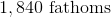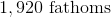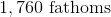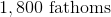Explanation:

Multiply 2 miles by 5,280 feet per mile byfathoms per foot: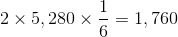fathoms.

Example Question #12 : Ratio And Proportion

A class hasgirls andboys. What is the ratio of girls to boys?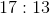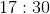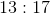Explanation:

The question asks to compare the number of girls to the number of boys. The ratio is.

Example Question #17 : Ratio And Proportion

If the ratio of boys to girl in a class is 2:3 and there are 18 boys in the class, how many girls are there?Explanation:

Since you know the ratio and the number of boys, you can set up a proportion from that ratio: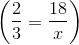.

Since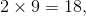you can multiply the denominator by 9 as well. Therefore,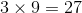and there are 27 girls in the class.

Example Question #18 : Ratio And Proportion

In a pet shop, there are 21 animals. There are 7 hamsters and 2 dogs. There are also equal numbers of cats, rabbits, and guinea pigs. What is the ratio of hamsters to guinea pigs?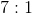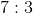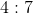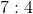Explanation:

In a pet shop, there are 21 animals. Since there are 7 hamsters and 2 dogs, this leaves 12 animals that are neither a hamster nor a dog.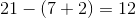Given that there are also equal numbers of cats, rabbits, and guinea pigs, this means that the remaining number of animals must be divided by 3 to find the number of each species.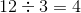There are 4 cats, 4 rabbits, and 4 guinea pigs.

The ratio of hamsters to guinea pigs is therefore 7:4 because there are 7 hamsters and 4 guinea pigs.

Example Question #19 : Ratio And Proportion

Mike invited forty friends from his current school, fifteen of whom are girls, to his birthday party. He wants the ratio of girls to boys at the party to be three to two, so he decides to invite some girls from his old school. How many girls will he need to invite, assuming he does not invite anyone else?Explanation:

So far, there are 15 girls and, counting Mike himself,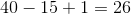boys. For the ratio of girls to boys to be 3 to 2, the number of girls must betimes the number of boys, or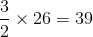girls.

15 girls have been invited already, so Mike needs to invite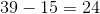more girls.

Example Question #20 : Ratio And Proportion

Sharon is having a birthday party. So far, she has invited twenty of her friends from school, nine of whom are girls. She wants to make the ratio of boys to girls at the party two to one. If she decides to add her two cousins, both boys, to the guest list, and no other girls, how many more boys does she need to invite?For there to be a two-to-one boy-to-girl ratio, Sharon must have twice as many boys as girls. She will need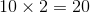boys total, so she will need to invite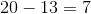more boys.